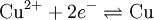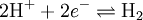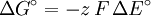# What is electrochemical series

## Electrochemical series

The Electrochemical series is a listing of redox pairs according to their standard electrode potential (redox potential under standard conditions). Especially with metals it will Redox series called. In this row, the oxidized and reduced forms, as well as the number of electrons transferred and the standard potential of a redox couple are listed next to each other. The individual redox pairs are sorted according to either increasing or decreasing standard electrode potential. Every redox reaction can be described by two pairs and the direction of reactions can be predicted from the electrochemical series.

### Metals

In the case of metals, the metal itself and its associated ion form a redox couple. For exampleCu is the reduced form ("Red") and Cu2+ the oxidized form ("ox"). The redox potential is a measure of the readiness of the ions to accept the electrons. The ions of the noble metals accept electrons more readily than the ions of base metals, which is why the redox potential of the Cu / Cu under standard conditions2+Pair with +0.35 V is clearly more positive than that of Zn / Zn2+-Pair with −0.76 V. And that in turn means that Zn belongs to the less noble metals and is a stronger reducing agent, i.e. its reactant is reduced and itself oxidized and releases electrons.

(“Under standard conditions” means that the concentration - more precisely: activity - of the ions must be 1 mol / l so that the redox potential assumes the values ​​in the table. This restriction is necessary because equilibrium reactions are involved. According to LeChatelier's principle, a A larger amount of metal ions also means a greater willingness to be reduced to the metal and therefore a higher redox potential. The Nernst equation describes this relationship mathematically.)

Redox potentials themselves cannot be measured. In contrast, the difference between two electrode potentials can be measured. An electrode under standard conditions is simply realized by immersing a metal in a solution that contains its ions in a concentration of 1 mol / l. If two such electrodes are connected in an electrically conductive manner (ion bridge), a galvanic cell is created and a voltage can be measured between the metals. This voltage is equal to the difference between the standard electrode potentials that belong to the redox pairs in the electrode spaces and are tabulated in the electrochemical series of voltages. For the example of the combination of the redox pairs Cu / Cu2+ and Zn / Zn2+ creates a Daniell element with a voltage of 1.11 V.

### Ion / gas electrodes (normal hydrogen electrode)

Gaseous hydrogen and proton are also a redox couple:Electrodes for redox pairs with gaseous substances are made by adding an inert metal (Pt) to a 1 mol / l solution of the ions (H.+) submerged and from the associated gas (H2) is washed around at a pressure of 1 bar. In a special case, a normal hydrogen electrode is created. This electrode is easy to set up and provides a constant, reproducible potential. Since the redox couple H2/H+ also describes the effect of acids (it always appears when metals dissolve in acids: e.g. Mg + 2H+ → Mg2+ + H2), the standard potential of the normal hydrogen electrode has been defined as zero for practical reasons.

All other standard potentials are therefore the voltages that are measured in a galvanic cell when the normal hydrogen electrode on the left and the electrode of the redox pair on the right are connected. (Each under standard conditions!)

### Applications

The electrochemical series of voltages allows the calculation of the maximum voltages that batteries and accumulators can deliver. Conversely, these are the voltages that must be applied at least to drive electrolysis or to charge the batteries.

It is also possible to calculate the direction and strength of the reaction. If two redox pairs are mixed in a reaction solution, the reduction will take place for the pair with the higher redox potential and the oxidation for the pair with the lower redox potential. If you dive z. B. a zinc sheet in a CuSO4Solution, zinc is oxidized due to its lower redox potential (−0.76 V) and goes into solution as zinc ions, whereas at the same time copper ions (+0.52 V) are reduced and form a copper coating on the zinc -Separate sheet metal. (This frequently cited example disregards the requirement for standard conditions. This is also how a copper sheet that is inserted into a ZnSO4- Dip the solution, coat a little with zinc, because initially there is no zinc and the Cu2+-Concentration are zero. The effect can be calculated with the Nernst equation, but it is immeasurably small, so that the example has a certain justification.) A measure of the strength of the reaction is the Gibbs energy (free enthalpy) of the associated reaction, which according tocan be calculated. Are in it z the number of electrons exchanged, F. = 96,485 C mol−1 the Faraday constant and ΔE. ° the difference between the standard potentials.

The reduced form of a redox couple with a very negative standard potential is a very strong reducing agent because it tends to donate electrons (e.g. sodium). In contrast, the oxidized form of a redox couple with a very positive standard potential is a strong oxidizing agent (e.g. fluorine as the strongest known oxidizing agent, i.e. with the highest standard potential) because it tends to accept electrons. The electrochemical series is thus a listing of oxidizing agents according to oxidation strength or, at the same time, a reverse listing of reducing agents according to reducing strength.

In addition, the electrochemical series contains a gradation of metals (“very noble metal”, “noble metal”, “less noble metal”, “base metal”, “very base metal”) according to their efforts to be oxidized in acids. The standard potentials of the noble metals have a positive sign, while those of the base metals have a negative sign. The base metals therefore dissolve in acids, because acids H+ contain. (The arguments for example Zn / Cu apply analogously.)

### Electrochemical series

(Standard potentials at 25 ° C; 101.3 kPa; pH = 0; ion activities = 1)

 oxidized form + z e− ⇌ reduced form Standard potential E. ° Element in the redox couple, its oxidation state changes Fluorine (F) F.2 + 2e− ⇌ 2 F− +2.87 V Sulfur (S) S.2O82− + 2e− ⇌ 2 SUN42− +2.00 V. Oxygen (O) H2O2 + 2 H.3O+ + 2e− ⇌ 4 H2O +1.78 V Gold (Au) Au+ + e− ⇌ Au +1.69 V Gold (Au) Au3+ + 3e− ⇌ Au +1.42 V Gold (Au) Au2+ + 2e− ⇌ Au+ +1.40V Chlorine (Cl) Cl2 + 2e− ⇌ 2Cl− +1.36V Oxygen (O) O2 + 4 H.3O+ + 4e− ⇌ 6 H2O +1.23V Platinum (Pt) Pt2+ + 2e− ⇌ Pt +1.20 V Bromine (Br) Br2 + 2e− ⇌ 2Br− +1.07V Mercury (Hg) Ed2+ + 2e− ⇌ Ed +0.85 V Silver (Ag) Ag + + e− ⇌ Ag +0.80 V Iron (Fe) Fe3+ + e− ⇌ Fe2+ +0.77 V Iodine (I) I.2 + 2e− ⇌ 2I− +0.53 V Copper (Cu) Cu+ + e− ⇌ Cu +0.52 V Iron (Fe) [Fe (CN)6]3− + e− ⇌ [Fe (CN)6]4− +0.361 V Copper (Cu) Cu2+ + 2e− ⇌ Cu +0.34 V. Copper (Cu) Cu2+ + e− ⇌ Cu+ +0.16 V Tin (Sn) Sn4+ + 2e− ⇌ Sn2+ +0.15 V. Hydrogen (H2) 2H+ + 2e− ⇌ H2 0 Iron (Fe) Fe3+ + 3e− ⇌ Fe −0.04 V Lead (Pb) Pb2+ + 2e− ⇌ Pb −0.13 V Tin (Sn) Sn2+ + 2e− ⇌ Sn −0.14 V Nickel (Ni) Ni2+ + 2e− ⇌ Ni −0.23 V Cadmium (Cd) CD2+ + 2e− ⇌ Cd −0.40 V Iron (Fe) Fe2+ + 2e− ⇌ Fe −0.41 V Sulfur (S) S. + 2e− ⇌ p2− −0.48 V Nickel (Ni) NOK2 + 2 H.2O + 2e− ⇌ Ni (OH)2 + 2 OH− −0.49 V Zinc (Zn) Zn2+ + 2e− ⇌ Zn −0.76 V water 2 H2O + 2e− ⇌ H2 + 2 OH− −0.83 V Chromium (Cr) Cr2+ + 2e− ⇌ Cr −0.91 V Niobium (Nb) Nb3+ + 3e− ⇌ Nb −1.099 V Vanadium (V) V.2+ + 2e− ⇌ V −1.17 V Manganese (Mn) Mn2+ + 2e− ⇌ Mn −1.18 V Titanium (Ti) Ti3+ + 3e− ⇌ Ti −1.21 V Aluminum (Al) Al3+ + 3e− ⇌ Al −1.66 V Titanium (Ti) Ti2+ + 2e− ⇌ Ti −1.77 V Beryllium (Be) Be2+ + 2e− ⇌ Be −1.85 V Magnesium (Mg) Mg2+ + 2e− ⇌ Mg −2.38 V Sodium (Na) N / A+ + e− ⇌ Well −2.71 V Calcium (Ca) Approx2+ + 2e− ⇌ Approx −2.76 V Barium (Ba) Ba2+ + 2e− ⇌ Ba −2.90 V Potassium (K) K+ + e− ⇌ K −2.92 V Lithium (Li) Li+ + e− ⇌ Li −3.05 V

### literature

• Handbook of Chemistry and Physics, CRC press, 1995 Electrochemical Series
• Inorganic Chemistry, Buchners Verlag, 1972
• Elements Chemistry II, Klett Verlag, 2000